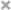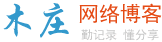# 如何使用html实现流星雨的效果（代码）

 12345678910111213141516171819202122232425262728293031323334353637383940414243444546474849505152535455565758596061626364656667686970717273747576777879808182838485868788899091929394959697 ```<``html``>``    ``<``head``>``        ``<``meta` `charset``=``"GB2312"` `/>``        ``<``title``>流星雨``        ``<``meta` `name``=``"keywords"` `content``=``"关键词，关键字"``>``        ``<``meta` `name``=``"description"` `content``=``"描述信息"``>``        ``<``style``>``            ``body {``                ``margin: 0;``                ``overflow: hidden;``            ``}``        ````    ```` ` `    ``<``body``>`` ` `        ````        ``<``canvas` `width``=``400` `height``=``400` `style``=``"background:#000000;"` `id``=``"canvas"``>`` ` `        ````        ``<``script``>``                    ` `            ``//获取画板``            ``//doccument 当前文档``            ``//getElement 获取一个标签``            ``//ById 通过Id名称的方式``            ``//var 声明一片空间``            ``//var canvas 声明一片空间的名字叫做canvas``            ``var canvas = document.getElementById("canvas");``            ``//获取画板权限 上下文``            ``var ctx = canvas.getContext("2d");``            ``//让画板的大小等于屏幕的大小``            ``/*``                ``思路：``                    ``1.获取屏幕对象``                    ``2.获取屏幕的尺寸``                    ``3.屏幕的尺寸赋值给画板``            ``*/``            ``//获取屏幕对象``            ``var s = window.screen;``            ``//获取屏幕的宽度和高度``            ``var w = s.width;``            ``var h = s.height;``            ``//设置画板的大小``            ``canvas.width = w;``            ``canvas.height = h;`` ` `            ``//设置文字大小 ``            ``var fontSize = 14;``            ``//计算一行有多少个文字 取整数 向下取整``            ``var clos = Math.floor(w/fontSize);``            ``//思考每一个字的坐标``            ``//创建数组把clos 个 0 （y坐标存储起来）``            ``var drops = [];``            ``var str = "qwertyuiopasdfghjklzxcvbnm";``            ``//往数组里面添加 clos 个 0``            ``for(var i = 0;i<``clos``;i++) {``                ``drops.push(0);``            ``}`` ` `            ``//绘制文字``            ``function drawString() {``                ``//给矩形设置填充色``                ``ctx.fillStyle``=``"rgba(0,0,0,0.05)"``                ``//绘制一个矩形``                ``ctx.fillRect(0,0,w,h);`` ` `                ``//添加文字样式``                ``ctx.font` `= ``"600 "``+fontSize+"px 微软雅黑";``                ``//设置文字颜色``                ``ctx.fillStyle` `= ``"#ff2d2d"``;`` ` `                ``for(var ``i` `= ``0``;ih&&Math.random()>0.99){``                        ``drops[i] = 0;``                    ``}``                    ``drops[i]++;``                ``}``                    ` `            ``}``            ``//定义一个定时器，每隔30毫秒执行一次``            ``setInterval(drawString,30);``        ````    `````

HTML5梦幻之旅――炫丽的流星雨效果实现过程_html5教程技巧

html在图片上实现下雨效果_html/css_WEB-ITnose

Html中url什么意思？怎么用？

Html如何提高页面的加载速度

Html如何设置字体文字的倾斜效果？（代码详解）

Html的特殊字符-css3 content:"特殊符号"应该如何使用

onclick在Html中用法是什么

Html怎么让图片居中

Html表格中的th表头内容怎么居中？th表头标签align属性的具体介绍

Html article标签怎么用

Html colgroup标签怎么用### 评论

• 欢迎访问木庄网络博客
• 可复制：代码框内的文字。
• 方法：Ctrl+C。